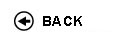Politecnico di Torino
Academic Year 2009/10
15BCGFL
Geometry
1st degree and Bachelor-level of the Bologna process in Environmental Engineering - Torino
 Teacher Status SSD Les Ex Lab Tut Years teaching Tedeschi Giulio 72 45 0 0 8
 SSD CFU Activities Area context MAT/03 10 A - Di base Matematica, informatica e statistica
 Objectives of the course This course gives the students skills for problem analysis (hypothesis, thesis, demonstration). Linear algebra and analytical geometry are studied, connected to Mathematical analysis I and II. Expected skills Comprehension of liner algebra and skills in using it in problem solving. Good skills in solving analitical and differential geometry problems, explaining the methods used. Prerequisites Real and complex numbers, trigonometric calculus, simple and quadratic equations and inequalities, differential calculus in one variable. Syllabus 1. Ordinary space vectors and their operations (sum; product). 2. Vectorial spaces in real and complex field. Spaces with a finite dimension. 3. Matrix and their operations. Reduced matrix, reduction method. 4. Linear systems. Rouchč-Capelli's theorem. Reductionmethod. 5. Linear applications. Linear applications in spaces with finite dimension. 6. Eigenvectors and eigenvalues. Simple endomorphisms. 7. Euclidean spaces and quadratic forms. 8. Plane analytical geometry. Etc. 9. Analytical geometry in space. Lines, planes, spheres, circumferences, curves, space surface, etc. 10. Differential calculus in many variables. Partial and directional derivative, gradient, divergence, rotor, differential, Taylor's formula. Etc. 11. differential geometry of curves and surface. Check availability at the library Programma provvisorio per l'A.A.2005/06© Politecnico di Torino
Corso Duca degli Abruzzi, 24 - 10129 Torino, ITALY